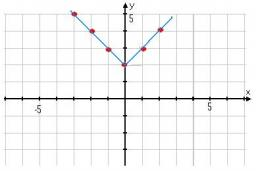# Inequalities: 4229

Find the number of all integers x that satisfy the following two inequalities:
| x + 2 | <11 a | x-4 |> = 3

n =  16

### Step-by-step explanation:Did you find an error or inaccuracy? Feel free to write us. Thank you!

Showing 1 comment:
Math student
Does the point (7, –3) satisfy the equation y = x2 − 6x − –2?

Tips for related online calculators
Do you have a linear equation or system of equations and looking for its solution? Or do you have a quadratic equation?